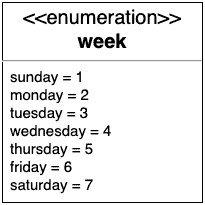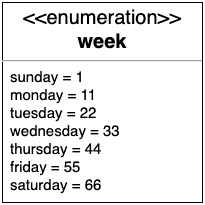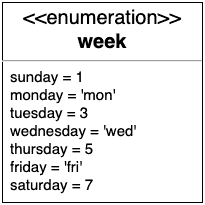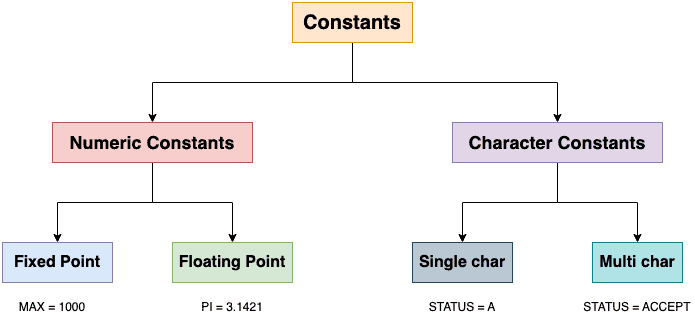## 1. Introduction

In this tutorial, we’ll study enumerations and constants. We’ll also point out when to use enumeration and when to adopt constants.

## 2. Enumerations

An enumeration (or enum) is a user-defined data type or UDT specified as a name-value set. In it, each value ​​represents an immutable constant (1, 2, etc.).

We also call it an enumerated type because we list each of the values while defining it.

Most of the time, we use enums for a smaller set of related variables that help us define and group a set of constants (integers, decimal numbers, strings, and so on). Most programming languages allow defining user-defined types, but each of these is mapped to the basic datatypes. such as integer, character, floating-point, string, boolean, and pointer types.

### 2.1. Example

Let’s understand enumeration with an example. Here, let’s say we want to represent a week and use it in our source code as an enumeration:So, the week is an enum with seven possible values starting with sunday, to which we assigned constant 1. Thereafter, the system automatically assigns each value a constant that is obtained by adding 1 to the last value.

The values need not be consecutive:### 2.2. Constant Types

An enum comprises names that are logically related and that we can consider to constitute the same type. So, we don’t make enumerations based on the data types of the underlying values.

Therefore, all the values we map the names to may or may not have the same data type. For example, we can define the enumeration of the week by giving different values to each of its values:Enumerations offer us the best way to define a small set of named values.

They help us make our code more structured and readable. Then, enums give us more type safety because we can type check an enum that we pass as an argument to functions, etc. This way, we reduce syntax and various manual errors in our code.

Enumerations also make our code forward-compatible.  That means that we can make a change only at the place where we have defined an enum, and the change will be propagated throughout the code.

Also, in most if not all languages, an enumeration allows us to iterate over its values in the order in which we enumerate them while defining it.

## 3. Constants

Constants are fixed single-value literals that we define once but can use at different places in different files throughout the program.

We can have constants of any data type. A constant is similar to a variable as we use both of these for storing values. However, we can’t modify a constant after defining it. In contrast, we can change a variable’s value as many times as we need.

We use constants to store a single value only and not a range as in the case of an enum.

### 3.1. Example

For instance, can use a constant PI to denote the math term. We assign it a constant literal value of 3.14159. Later, we can use PI instead of the value 3.14159 in our code that calculated the area of a circle with a given radius:This shows why constants are useful. If we later want to add more precision to PI, we only need to update the constant. If we didn’t use the constant, we’d have to go over our code and manually update each calculation in which 3.14159 participated as a literal.

### 3.2. Types of Constants

Most programming languages have two types of constants: the numeric and the character ones. We further divide the former into fixed-point constants (integers) and floating-point constants (decimal numbers). Similarly, we classify character constants as single-character or multi-character constants (the latter we also call strings):Now, let’s enumerate some advantages of constants.

Constants are immutable during the execution of our program. They offer us a very simple way to define a literal we can later use throughout our code without the fear of it being modified.

Constants make it easier to maintain our code. We define them once and then can use them multiple times. This way, we save time and reduce the code footprint.

Thirdly, constants save our system memory since we generally store them in a read-only section of memory (ROM) and not in the main memory (RAM). Since ROM access is faster than RAM access, constants also speed up our code.

Furthermore, constants make our documentation task easier since we define them in a single place. That way, we can update only one place and trigger software recompilation to generate documentation. So, even though constants aren’t changeable during execution, we can easily change them when coding, and those changes will be visible in the entire codebase.

## 4. Enums vs. Constants

We can replace an enumeration havingvalues withdifferent constants. But, we’ll find it very hard to iterate through all theseconstant values as compared to allvalues of a single enumeration.

The following table shows a quick comparison between constants and enums:## 5. Conclusion

In this article, we have studied enumerations and constants from a programming context. We use enumerations to store a finite set of named values whereas we use constants to store a single value.